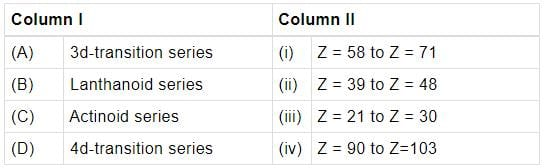# NCERT Based Test: Electronic Configuration of Elements & The Periodic Table

## 5 Questions MCQ Test NCERTs for NEET | NCERT Based Test: Electronic Configuration of Elements & The Periodic Table

Description
Attempt NCERT Based Test: Electronic Configuration of Elements & The Periodic Table | 5 questions in 5 minutes | Mock test for NEET preparation | Free important questions MCQ to study NCERTs for NEET for NEET Exam | Download free PDF with solutions
QUESTION: 1

### Anything that influences the valence electrons will affect the chemistry of the element. Which one of the following factors does not affect the valence shell?

Solution:

Nuclear mass does not affect the valence shell because nucleus consists of protons and neutrons. Whereas proton, i.e. nuclear charge affects the valence shell but neutrons do not. Thus, option (c) is wrong.

QUESTION: 2

### Which of the following elements shown as pairs with their atomic numbers belong to the same period?

Solution:

Z = 12, 1s2, 2s2, 2p6, 3s2 (n = 3)
Z = 17, 1s2 2s2 2p6 3s2 3p5 (n = 3)

QUESTION: 3

### To which group,an element with atomic number 88 will belong?

Solution:

Z = 88, [Rn] 7s2, hence belongs to group 2.

QUESTION: 4

Match the column I with column II and mark the appropriate choice.Solution:

(A) 3d-transition series, Z = 21 (Sc) to Z=30 (Zn)
(B) Lanthanoid series, Z = 58 (Ce) to Z = 71 (Lu)
(C) Actinoid series, Z = 90 (Th) to Z = 103 (Lr)
(D) 4d-transition Z = 39 (Y) to Z = 48 (Cd) series,

QUESTION: 5

An element has atomic number 79. Predict the group and period in which the element is placed.

Solution:

Z = 79 [Xe]4f145d10.6s1 (n = 6)
As n = 6, the element belongs to 6th period.
Group = ns + (n - I)d, I + 10 = 11Use Code STAYHOME200 and get INR 200 additional OFF Use Coupon Code